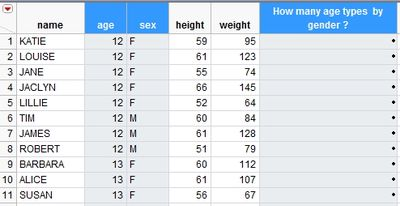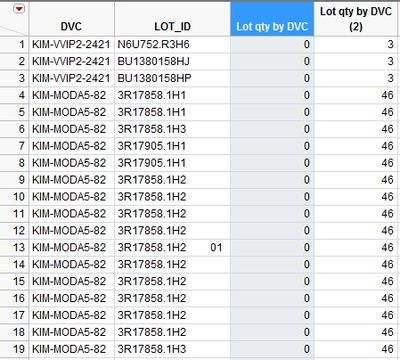Choose Language Hide Translation Bar
Highlighted

## About formula : "How many (categorical data) by column ?

Hi there~

Could you do me a favor?

I want to know

"How many (categorical data) by column

without calculation of repeated data?

=========================================================

for example

Open( "\$SAMPLE_DATA/Big Class.jmp" );

I want to know how many (:age) types by (:sex)?

I want to know that function by using formula only1 ACCEPTED SOLUTION

Accepted Solutions
Highlighted

## Re: About formula : "How many (categorical data) by column ?

Oh, I forgot that Col Number() only works for numeric columns as first argument.

Here two suggestion for a column formula solution (and not by JSL-script ):

Use a numeric column that's known to not have any missing values, e.g.

Col Number( :TRANS_TIME, :DVC )

Or if each DVC data always ends with a unique four digit number (as in the posted table) this would give the same result:

Col Number( Num( Right( :DVC, 4 ) ), :DVC )

4 REPLIES 4
Highlighted

## Re: About formula : "How many (categorical data) by column ?

Use this Statistical function:

Col Number( :age, :sex )

The second argument is the By-bariable. In formula editor the optional second argument (and third etc) is activated by selecting the first argument and clicking on ^ (insert).

Highlighted

## Re: About formula : "How many (categorical data) by column ?

Dear MS,

I really appreciate with ur idea.

But I still cannot solve this problem.

b'cus my data is a little bit more complicate.

There are repeated same data in the "name" column,

.. therefore...  Col Number( :age, :sex ) is not working..

This is actual sample..

when I use this

Col Number(:LOT_ID, :DVC)

but it is not working,,, the result shows "0" only  like as below.

I think ... it is because of the same data in 'LOT_ID' column

but i still want to calculate it.I soIve it, by using other column data "time"  like as below.

Col Sum(If(Col Minimum(:TRANS_TIME, :LOT_ID) == :TRANS_TIME, 1, 0), :DVC)

But I still want to solve it    without the other data.

Could you give me  the other idea?  I need ur help.. MS

Highlighted

## Re: About formula : "How many (categorical data) by column ?

Oh, I forgot that Col Number() only works for numeric columns as first argument.

Here two suggestion for a column formula solution (and not by JSL-script ):

Use a numeric column that's known to not have any missing values, e.g.

Col Number( :TRANS_TIME, :DVC )

Or if each DVC data always ends with a unique four digit number (as in the posted table) this would give the same result:

Col Number( Num( Right( :DVC, 4 ) ), :DVC )

Highlighted

## Re: About formula : "How many (categorical data) by column ?

Oh~ MS~!

I truly appreciate you taking the time to answer my questions!